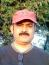# Questions 27 & 31 of Oliverlehmann

Hello everyone,

i need help with questions 27 and 31 of Oliverlehmann.

thanks.

http://www.oliverlehmann.com/pmp-self-test/75-free-questions.htm

### Check### Q27) Standard deviaitoin for

Q27) Standard deviaitoin for ranges always you need calculate the SD for individual P-O/6

Then Calculate the variance for each of the SD calculated, ( P-O/6)^2

Then add up the variances to find the final value of SD

SD 24-12/6= 12/6=2, variance=square of 2=4
SD 14-8/6=6/6=1, variance=1
SD 27-15/6=12/6=2, variance =4
SD 28-10/6=18/6=3, variance=9
SD 35-17/6=18/6=3, variance=9

now add up all variances= 4+1+4+9+9=27
now take sq root of 27=5.2

Regards

CN Patil### Q31) Act1=20 days Act2=10

Q31)

Act1=20 days

Act2=10 days

Act3=5 days with FF-2 days

Act 4=6 days with FS+3

Now since we have FF relationship  and lead of 2days, will consider Act3 will finish along with Act2 and since there is lead of 2 days, then it should deducted from next Act4

so, Act1 (20days) + Act2 (10days)+ Act4 (6days) +3 days for lag - 2 days for lead

20+10+6+3-2= 37 days

Note Act3 do not coem into picture for calculation but it's lead time is considered for substracting.

Regards

CN Patil

### Is does this always apply

When they say FF between A and B, this means for B to finish (completion of the successor), A must finish first (depends upon completion of the predecissor).

So between Act2 and 3 there is a FF realtionship with -2 (i understood it as a lag of 2 days).

Your answer is the right one but also very simple. Just add the numbers....### Thanks

Thanks both of you. Appreciate your help.

### Q31, bar chart

for this question, draw a bar(gantt) chart.

It greatly simplifies the problem.